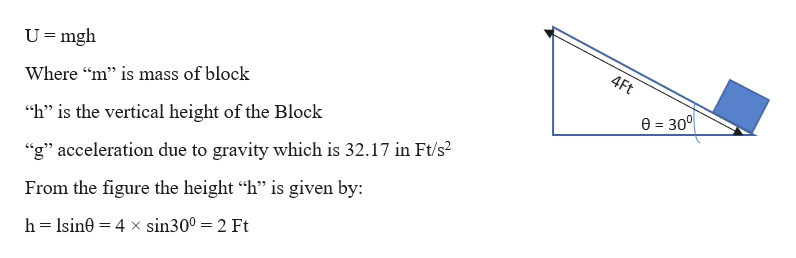A woman exerts a horizontal force of 10 pounds on a box as she pushes it up a ramp that is 4 feet long and inclined at an angle of 30 degrees above the horizontal.Find the work done on the box.

Question

A woman exerts a horizontal force of 10 pounds on a box as she pushes it up a ramp that is 4 feet long and inclined at an angle of 30 degrees above the horizontal.

Find the work done on the box.

Step 1

Given information:

The inclination of the ramp (θ) = 300

The length of the ramp (l) = 4 feet

The force with which the women pushes the block (F) = 10 pounds

Let “m” be the mass of the block

Let “W” be the work done

Step 2

The work done by the women is given by the change in potential energy of the block

Change in potential energy is given by:help_outlineImage TranscriptioncloseU mgh 4Ft Where "m" is mass of block e 300 "h" is the vertical height of the Block "g" acceleration due to gravity which is 32.17 in Ft/s2 From the figure the height "h" is given by: h Isin0 4 x sin300 = 2 Ft fullscreen
Step 3

The work done by women is:

W = m × 32.17 × 2 &...

Want to see the full answer?

See Solution

Want to see this answer and more?

Our solutions are written by experts, many with advanced degrees, and available 24/7

See Solution
Tagged in

Work,Power and Energy